## Screenshots

•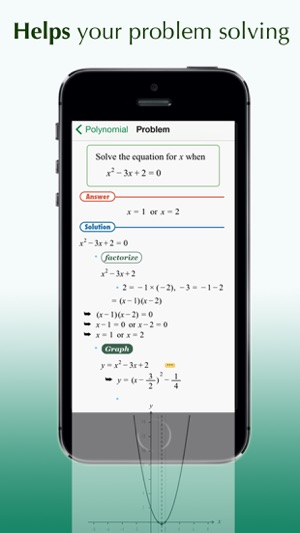•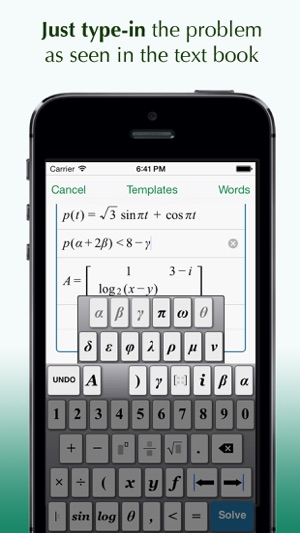•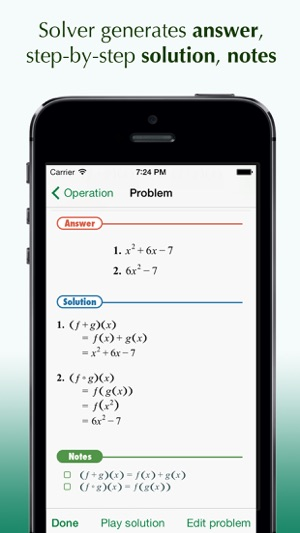•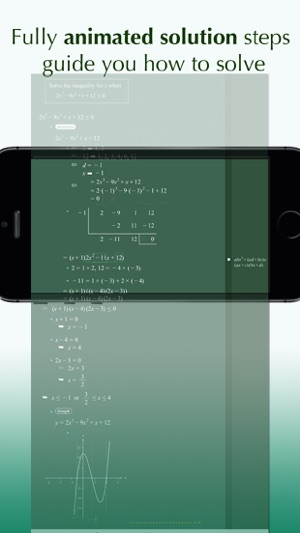•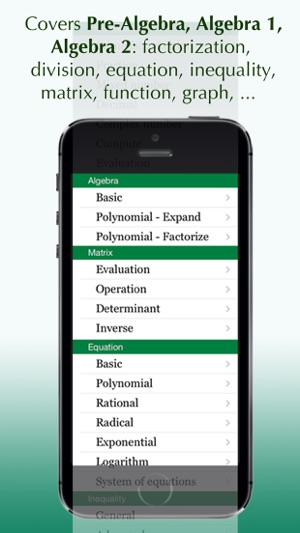## Description

This app is distributed for Educational Institutions. Evaluation users and students are recommended to try the free version of the app: either FX Math Solver or FX Algebra Solver.

FX Algebra for Education is a math tutoring and assistant tool for middle and high-school students, and distinguished from other math apps, in the following aspect:

- For user typed-in problems, this app provides both static and dynamic(-animated) form of answers and solutions, so students can easily follow the problem solving steps applied in the derivation of answer.

- The internal rule-base of the problem solving server is based on the know-how and heuristics of real human problem solving experts, so compared to other apps based on algorithmic problem solving server, the solution generated by this app looks readable and familiar to students.

- To help students type-in problem expressions easily, this app embeds visual math expression editor, similar to desktop word-processing programs.

FX Algebra for Education is a comprehensive math software, based on an automatic mathematical problem solving engine, including:

- Over 1,200 sample math problems and fully animated solution steps
- Scientific calculator supported
- Graphing calculator supported
- Automatic problem solving and generation of fully animated step-by-step procedures for problems typed in by users
- User friendly math problem expression editor (WYSIWYG mode)

The problem solving server can solve problems at the level of Pre-Algebra, Algebra1, Algebra2, and Calculus courses:

- Number operations: add, subtract, multiply, divide
- Prime factorization
- Mixed number
- Complex number

- Basic expression simplification:
- polynomial - factorization, long-division
- rational
- exponential and log/ln
- sin, cos, tan, ..
- Matrix
- Equations, system of equations
- Inequalities

- Function

- Graphing

## What’s New

Version 1.1

This app has been updated by Apple to use the latest Apple signing certificate.

bug fix

## Information

Provider
Euclidus Inc
Size
40.6 MB
Category
Education
Compatibility

Requires iOS 7.1 or later. Compatible with iPhone, iPad and iPod touch.

Languages

English

Age Rating
4+
Price
USD 7.99

## Supports

•